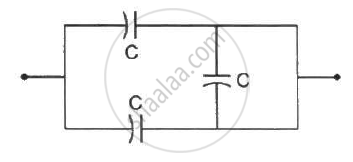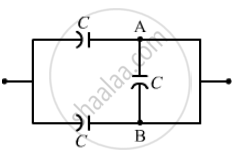Department of Pre-University Education, Karnataka course PUC Karnataka Science Class 12
Share

# The Equivalent Capacitance of the Combination Shown in Figure (31-q1) is - Physics

ConceptCapacitors and Capacitance

#### Question

The equivalent capacitance of the combination shown in the figure is _________ .• C

• 2 C

• C/2

• none of these.

#### Solution

2CSince the potential at point A is equal to the potential at point B, no current will flow along arm AB. Hence, the capacitor on arm AB will not contribute to the circuit. Also, because the remaining two capacitors are connected in parallel, the net capacitance of the circuit is given by

C_"eq" = C + C = 2C

Is there an error in this question or solution?

#### Video TutorialsVIEW ALL 

Solution The Equivalent Capacitance of the Combination Shown in Figure (31-q1) is Concept: Capacitors and Capacitance.
S